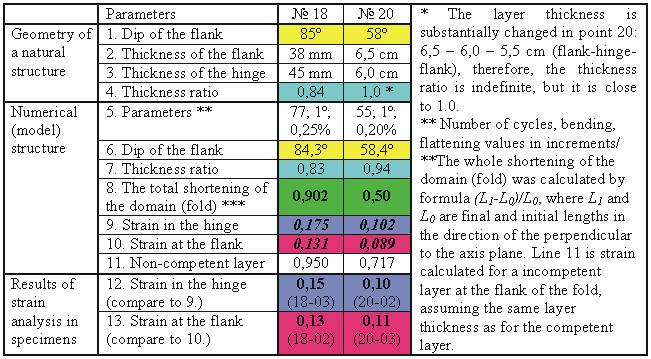last update

12/05/2009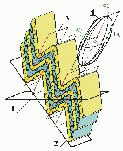Yakovlev Fedor

Home-page

FOLDING formation research

Guide:

about the study of folded structures of several scale levels into thin-layered flysch-like sediments in a Hinterland

II.     SEPARATE FOLDS AND STRAIN VALUE ESTIMATION

 4.  Intra-layer structures (rank 1) – since 2002. ·Description. Usual methods of strain-analysis used for intra-layer deformation study in specimens. Additional theoretic method is the model of multilayer folds formation which include the calculation of ellipses of deformation for hinge section (Fig. 1, into competent and incompetent layers) and for flank section (the same layers, total - 4 ellipses). This model was used for study of two folds of Caucasus (N18 and N20, fig. 2, 3), their specimens were studied in strain aspect also. A good concordance between natural fold shapes (table, points 1, 4) and model fold shapes (points 6,7) also as strains natural (points 12, 13) and models (9, 10) have been found. Total shortening values of models (point 8) were rather different with strain in specimens. It means that the model (Fig.1) allows us to spread correctly the strain analysis data to volume larger then specimens only, based on total fold shortening of model (point 8). Some information regarding this item has described in PhD thesis (Rus)  by Vyacheslav Voitenko. Details. ·Publications. (the same as in point 4 lower) The first publication of theoretic model was in (Yakovlev “Investigation of the processes…”, 2002 г. Text: 319-320, picture 319; PDF -R, N 12 in List-En); the program see in folder SOFT. The first application of method as comparison of model data with natural one was published in (Yakovlev, Voitenko, at al. “Regarding ratio of shortening deformations...”, 2003 г. PDF-R, see Full List). ·Materials. (the same as in point 6 of Guid lower) The program code (*.exe + explanation + test examples for study) may be send after your inquiry using e-mail. If you have difficulties in strain-analysis study, our expert Vyacheslav Voitenko may assistant you. Let me know about your interest by e-mail. ·Some ideas for future works. (the same as in point 4 lower) There is topic for diploma work about comparison of objects ranks 1 with 2. A student may find the competent layer in similar type fold in field, to collect measurements of geometrical parameters and to collect the set of specimens. Usual strain-analysis is used for 3D ellipsoid deformation calculation for specimens. The theoretic model and geometry of fold in competent layer is used for ellipsoid deformation prediction. Results of work are 1) Comparison of natural object and model, 2) Value of deformation for specimens and fold. Let me know about your interest.   * ----------------------------------------------------------- Fig. 1 (to right). There is the model of intra-layer strain in similar folds. Competent layer is deforming by multi-iteration calculations of 1) buckling (rotation for a flank) and 2) flattening (the same value for all 4 cases). Results are shown in cases 1 and 3 (K1 and K3) as ellipses and its orientations. The incompetent layer is deforming by multi-iteration of 1) “rotation and simple shearing along bedding” as analogy of buckling and 2) flattening. Results are shown in cases 2 and 4 (K2 and K4).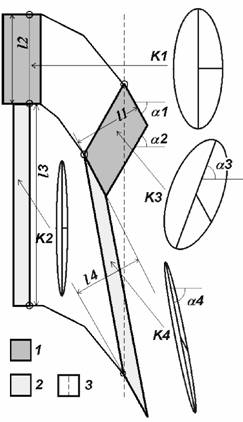Fig. 1. The model of intra-layer strain in similar folds. Total shortening value for the fold is К=0.396; ellipses shortening values are К1=0.643, К2=0.286, К3=0.668, К4=0.251; a1=61.4°, a2=–59.8°, a3=–69.8°, a4=78.7°; see also the explanation of model on left. 1 – competent layer; 2 – incompetent layer; 3 – axial plain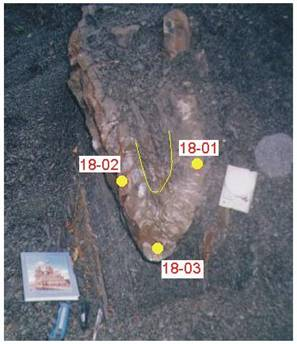Fig. 2.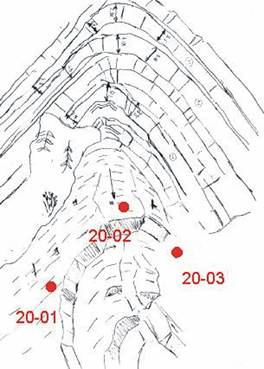Fig. 3. Table